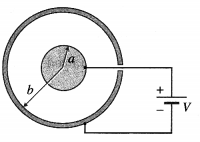Balbharti Maharashtra State Board 12th Physics Textbook Solutions Chapter 8 Electrostatics Textbook Exercise Questions and Answers.

## Maharashtra State Board 12th Physics Solutions Chapter 8 Electrostatics

1. Choose the correct option

i) A parallel plate capacitor is charged and then isolated. The effect of increasing the plate separation on charge, potential, capacitance respectively are
(A) Constant, decreases, decreases
(B) Increases, decreases, decreases
(C) Constant, decreases, increases
(D) Constant, increases, decreases
(A) Constant, decreases, decreases

ii) A slab of material of dielectric constant k has the same area A as the plates of a parallel plate capacitor and has thickness (3/4d), where d is the separation of the plates. The change in capacitance when the slab is inserted between the plates is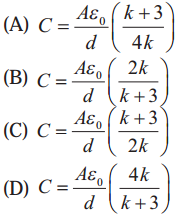(D) C = $$\frac{\varepsilon_{0} A}{d}\left(\frac{4 k}{k+3}\right)$$iii) Energy stored in a capacitor and dissipated during charging a capacitor bear a ratio.
(A) 1 : 1
(B) 1 : 2
(C) 2 : 1
(D) 1 : 3
(A) 1 : 1

iv) Charge + q and -q are placed at points A and B respectively which are distance 2L apart. C is the mid point of A and B. The work done in moving a charge +Q along the semicircle CRD as shown in the figure below is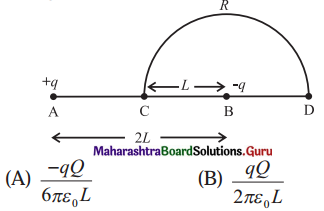(C) $$\frac{q Q}{6 \pi \varepsilon_{0} L}$$
(D) $$\frac{-q Q}{6 \pi \varepsilon_{0} L}$$
(A) $$-\frac{Q q_{1}}{6 \pi \varepsilon_{0} L}$$

v) A parallel plate capacitor has circular plates of radius 8 cm and plate separation 1mm. What will be the charge on the plates if a potential difference of 100 V is applied?
(A) 1.78 × 10-8 C
(B) 1.78 × 10-5 C
(C) 4.3 × 104 C
(D) 2 × 10-9 C
(A) 1.78 × 10-8 C

i) A charge q is moved from a point A above a dipole of dipole moment p to a point B below the dipole in equitorial plane without acceleration. Find the work done in this process.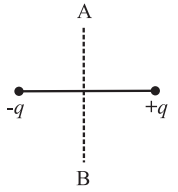The equatorial plane of an electric dipole is an equipotential with V = 0. Therefore, the no work is done in moving a charge between two points in the equatorial plane of a dipole.ii) If the difference between the radii of the two spheres of a spherical capacitor is increased, state whether the capacitance will increase or decrease.
The capacitance of a spherical capacitor is C = 4πε0$$\left(\frac{a b}{b-a}\right)$$ where a and b are the radii of the concentric inner and outer conducting shells. Hence, the capacitance decreases if the difference b – a is increased.

iii) A metal plate is introduced between the plates of a charged parallel plate capacitor. What is its effect on the capacitance of the capacitor?
Suppose the parallel-plate capacitor has capacitance C0, plates of area A and separation d. Assume the metal sheet introduced has the same area A.

Case (1) : Finite thickness t. Free electrons in the sheet will migrate towards the positive plate of the capacitor. Then, the metal sheet is attracted towards whichever capacitor plate is closest and gets stuck to it, so that its potential is the same as that of that plate. The gap between the capacitor plates is reduced to d – t, so that the capacitance increases.

Case (2) : Negligible thickness. The thin metal sheet divides the gap into two of thicknesses d1 and d1 of capacitances C1 = ε0A/d1 and C2 = ε0A/d2 in series.
Their effective capacitance is
C = $$\frac{C_{1} C_{2}}{C_{1}+C_{2}}=\frac{\varepsilon_{0} A}{d_{1}+d_{2}}=\frac{\varepsilon_{0} A}{d}$$ = C0
i.e., the capacitance remains unchanged.

iv) The safest way to protect yourself from lightening is to be inside a car. Justify.
There is danger of lightning strikes during a thunderstorm. Because trees are taller than people and therefore closer to the clouds above, they are more likely to get hit by lightnings. Similarly, a person standing in open ground is the tallest object and more likely to get hit by a lightning. But car with a metal body is an almost ideal Faraday cage. When a car is struck by lightning, the charge flows on the outside surface of the car to the ground but the electric field inside remains zero. This leaves the passengers inside unharmed.

v) A spherical shell of radius b with charge Q is expanded to a radius a. Find the work done by the electrical forces in the process.
Consider a spherical conducting shell of radius r placed in a medium of permittivity ε. The mechanical force per unit area on the charged conductor is
f = $$\frac{F}{d S}=\frac{\sigma^{2}}{2 \varepsilon}$$
where a is the surface charge density on the conductor. Given the charge on the spherical shell is Q, (σ = Q/πr2. The force acts outward, normal to the surface.

Suppose the force displaces a charged area element adS through a small distance dx, then the work done by the force is
dW = Fdx = ($$\frac{\sigma^{2}}{2 \varepsilon}$$ dS) dx
During the displacement, the area element sweeps out a volume dV = dS ∙ dx.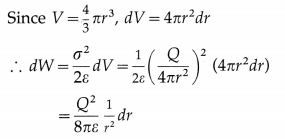Therefore, the work done by the force in expanding the shell from radius r = b to r = a is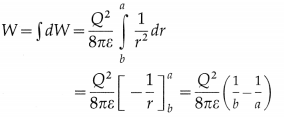This gives the required expression for the work done.Question 3.
A dipole with its charges, -q and + q located at the points (0, -b, 0) and (0 +b, 0) is present in a uniform electric field E whose equipotential surfaces are planes parallel to the YZ planes.
(a) What is the direction of the electric field E?
(b) How much torque would the dipole experience in this field?
(a) Given, the equipotentials of the external uniform electric field are planes parallel to the yz plane, the electric field $$\vec{E}=\pm E \hat{\mathrm{i}}$$ that is, $$\overrightarrow{E}$$ is parallel to the x-axis.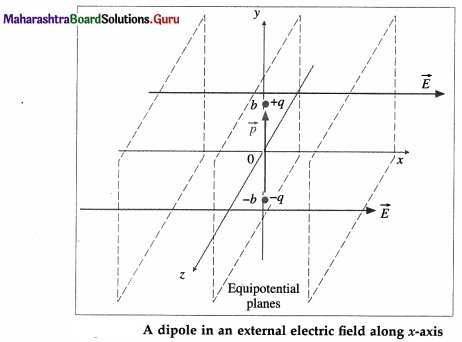(b) From above figure, the dipole moment, $$\vec{p}=q(2 b) \hat{\mathrm{j}}$$
The torque on this dipole,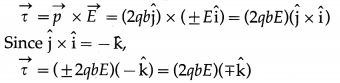So that the magnitude of the torque is τ = 2qbE.
If $$\vec{E}$$ is in the direction of the + x-axis, the torque $$\vec{\tau}$$ is in the direction of – z-axis, while if $$\vec{E}$$ is in the direction of the -x-axis, the torque $$\vec{\tau}$$ is in the direction of + z-axis.

Question 4.
Three charges – q, + Q and – q are placed at equal distance on straight line. If the potential energy of the system of the three charges is zero, then what is the ratio of Q : q?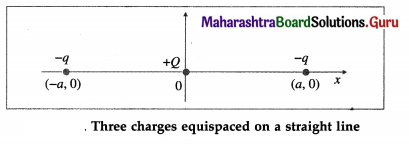In the above figure, the line joining the charges is shown as the x-axis with the origin at the + Q charge. Let q1 = +Q, and q2 = q3 = – q. Let the two – q charges be at (- a, 0) and (a, 0), since the charges are given to be equidistant.
∴ r21 = r31 = a and r32 = 2a
The total potential energy of the system of three charges is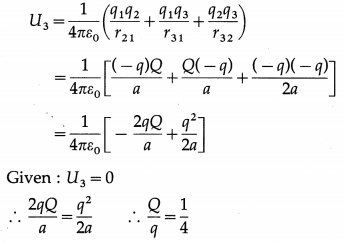This gives the required ratio.

Question 5.
A capacitor has some dielectric between its plates and the capacitor is connected to a DC source. The battery is now disconnected and then the dielectric is removed. State whether the capacitance, the energy stored in it, the electric field, charge stored and voltage will increase, decrease or remain constant.
Assume a parallel-plate capacitor, of plate area A and plate separation d is filled with a dielectric of relative permittivity (dielectric constant) k. Its capacitance is C = $$\frac{k \varepsilon_{0} A}{d}$$ …………. (1)
If it is charged to a voltage (potential) V, the charge on its plates is Q = CV.

Since the battery is disconnected after it is charged, the charge Q on its plates, and consequently the product CV, remain unchanged.

On removing the dielectric completely, its capacitance becomes from Eq. (1),
C’ = $$\frac{\varepsilon_{0} A}{d}=\frac{1}{k} C$$ ……………. (2)
that is, its capacitance decreases by the factor k. Since C’V’ = CV, its new voltage is
V’ = $$\frac{C}{C^{\prime}}$$ V = kV …………… (3)

so that its voltage increases by the factor k. The stored potential energy, U = $$\frac{1}{2}$$ QV, so that Q remaining constant, U increases by the factor k. The electric field, E = V/ d, so that E also increases by a factor k.Question 6.
Find the ratio of the potential differences that must be applied across the parallel and series combination of two capacitors C1 and C2 with their capacitances in the ratio 1 : 2, so that the energy stored in these two cases becomes the same.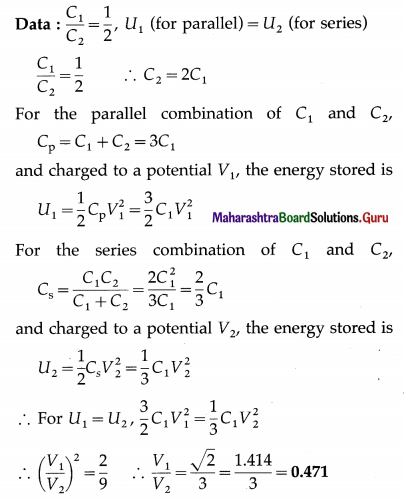This gives the required ratio.

Question 7.
Two charges of magnitudes -4Q and +2Q are located at points (2a, 0) and (5a, 0) respectively. What is the electric flux due to these charges through a sphere of radius 4a with its centre at the origin?
The sphere of radius 4a encloses only the negative charge Q1 = -4Q. The positive charge Q2 = +2Q being located at a distance of 5a from the origin is outside the sphere. Only a part of the electric flux lines originating at Q2 enters the sphere and exits entirely at other points. Hence, the electric flux through the sphere is only due to Q1.
Therefore, the net electric flux through the sphere = $$\frac{Q_{1}}{\varepsilon_{0}}=\frac{-4 Q}{\varepsilon_{0}}$$ . The minus sign shows that the flux is directed into the sphere, but not radially since the sphere is not centred on Q1.

Question 8.
A 6 µF capacitor is charged by a 300 V supply. It is then disconnected from the supply and is connected to another uncharged 3µF capacitor. How much electrostatic energy of the first capacitor is lost in the form of heat and electromagnetic radiation ?
Data: C = 6 µF = 6 × 10-6 F = C1, V = 300 V
C2 = 3 µF
The electrostatic energy in the capacitor
= $$\frac{1}{2}$$Cv2 = $$\frac{1}{2}$$(6 × 10-6)(300)2
= 3 × 10-6 × 9 × 104 = 0.27J
The charge on this capacitor,
Q = CV = (6 × 10-6)(300) = 1.8 mC
When two capacitors of capacitances C1 and C2 are connected in parallel, the equivalent capacitance C
= C1 + C2 = 6 + 3 = 9 µF
= 9 × 10-6F
By conservation of charge, Q = 1.8 C.
∴ The energy of the system = $$\frac{Q^{2}}{2 C}$$
= $$\frac{\left(1.8 \times 10^{-3}\right)^{2}}{2\left(9 \times 10^{-6}\right)}=\frac{18 \times 10^{-8}}{10^{-6}}$$ = 0.18 J
The energy lost = 0.27 – 0.18 = 0.09 JQuestion 9.
One hundred twenty five small liquid drops, each carrying a charge of 0.5 µC and each of diameter 0.1 m form a bigger drop. Calculate the potential at the surface of the bigger drop.
Data : n = 125, q = 0.5 × 10-6 C, d = 0.1 m
The radius of each small drop, r = d/2 = 0.05 m
The volume of the larger drop being equal to the volume of the n smaller drops, the radius of the larger drop is
R = $$\sqrt{n} r$$ = $$\sqrt{125}$$ (0.05) = 5 × 0.05 = 0.25 m
The charge on the larger drop,
Q = nq = 125 × (0.5 × 10-6) C
∴ The electric potential of the surface of the larger drop,
V = $$\frac{1}{4 \pi \varepsilon_{0}} \frac{Q}{R}$$ = (9 × 109) × $$\frac{125 \times\left(0.5 \times 10^{-6}\right)}{0.25}$$
= 9 × 125 × 2 × 103 = 2.25 × 106 V

Question 10.
The dipole moment of a water molecule is 6.3 × 10-30 Cm. A sample of water contains 1021 molecules, whose dipole moments are all oriented in an electric field of strength 2.5 × 105 N /C. Calculate the work to be done to rotate the dipoles from their initial orientation θ1 = 0 to one in which all the dipoles are perpendicular to the field, θ2 = 90°.
Data: p = 6.3 × 10-30 C∙m, N = 1021 molecules,
E = 2.5 × 105 N/C, θ0 = θ1 = 0°, θ = θ2 = 90°
W = pE(cos θ0 – cos θ)
The total work required to orient N dipoles is
W = NpE(cos θ1 – cos θ2)
=(1021)(6.3 × 10-30)(2.5 × 105)
= 15.75 × 10-4 J = 1.575 mJ

Question 11.
A charge 6 µC is placed at the origin and another charge -5 µC is placed on the y axis at a position A (0, 6.0) m.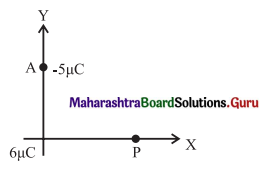(a) Calculate the total electric potential at the point P whose coordinates are (8.0, 0) m
(b) Calculate the work done to bring a proton from infinity to the point P. What is the significance of the sign of the work done ?
Data : q1 = 6 × 10-6 C, q2 = -5 × 10-6 C,
A ≡ (0, 6.0 m), P ≡ (8.0 m, 0), r1 = OP = 8 m, q = e = 1.6 × 10-19C, 1/4πε0 = 9 × 109 N∙m2/C2
r2 = AP = $$\sqrt{(8-0)^{2}+(0-6)^{2}}$$ = $$\sqrt{64+36}$$ = 10 m

(a) The net electric potential at P due to the system of two charges is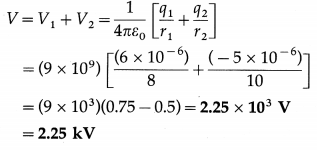(b) The electric potential V at the point P is the negative of the work done per unit charge, by the electric field of the system of the charges q1 and q2, in bringing a test charge from infinity to that point.
V = $$-\frac{W}{q_{0}}$$
∴ W = -qV= -(1.6 × 10-19)(2.25 × 103)
= -3.6 × 10-16 J= -2.25 keV
That is, in bringing the positively charged proton from a point of lower potential to a point of higher potential, the work done by the electric field on it is negative, which means that an external agent must bring the proton against the electric field of the system of the two source charges.

[Note : The potential V at a point is the work done per unit charge (Wext) by an external agent in bringing a test charge from infinity to that point. In the above case, the work done by an external agent will be positive. The question does not specify this.]Question 12.
In a parallel plate capacitor with air between the plates, each plate has an area of 6 × 10-3 m2 and the separation between the plates is 2 mm.
i) Calculate the capacitance of the capacitor.
ii) If this capacitor is connected to 100 V supply, what would be the charge on each plate?
iii) How would charge on the plates be affected if a 2 mm thick mica sheet of k = 6 is inserted between the plates while the voltage supply remains connected ?
Data: k = 1(air), A = 6 × 10-3 m2, d = 2
mm = 2 × 10-3 m,V = 100V, t = 2 mm = d, k1 = 6,
ε0 = 8.85 × 10-12 F/m
(i) The capacitance of the air capacitor, C0 = $$\frac{\varepsilon_{0} A}{d}$$
= $$\frac{\left(8.85 \times 10^{-12}\right)\left(6 \times 10^{-3}\right)}{2 \times 10^{-3}}$$
= 26.55 × 10-12 F = 26.55 pF

(ii) Q0 = C0V = (26.55 × 10-12)(100)
= 26.55 × 10-10 C = 2.655 nC

(iii) The dielectric of relative permittivity k1 completely fills the space between the plates (∵t = d), so that the new capacitance is C = k1C0.
With the supply still connected, V remains the same.
∴ Q = CV = kC0V = kQ0 =6(2.655 nF) = 15.93 nC
Therefore, the charge on the plates increases.
[Note: Ck1C0 = 6(26.55 pF)= 159.3 pF.]

Question 13.
Find the equivalent capacitance between P and Q. Given, area of each plate = A and separation between plates = d.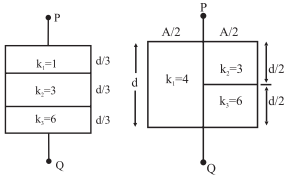(i) The capacitor in figure is a series combination of three capacitors of plate separations d/3 and plate areas A, with C1 filled with air (k1 = 1), C2 filled with dielectric of k2 = 3 and C3 filled with dielectric of k3 = 6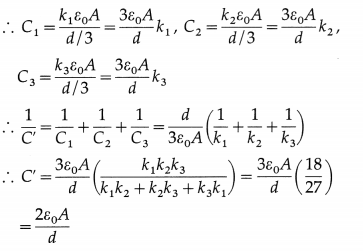(ii) In figure, a series combination of two capacitors C2(k2 = 3) and C3(k3 = 6), of plate areas A/2 and plate separations d/2, is in parallel with a capacitor C1 (k1 = 4) of plate area A/2 and plate separation d.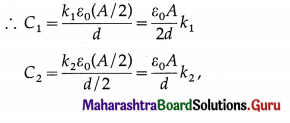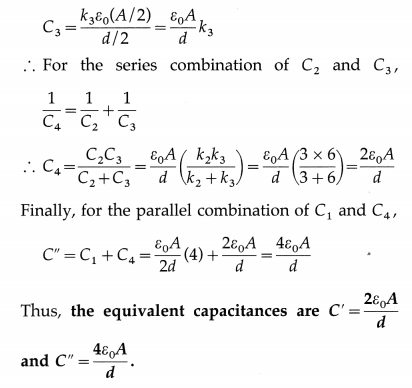12th Physics Digest Chapter 8 Electrostatics Intext Questions and Answers

Can you recall (Textbook Page No. 188)

Question 1.
What is gravitational Potential ?
We measure the gravitational potential energy U of a body (1) by assigning U = 0 for a reference configuration (such as the body at a reference level) (2) then equating U to the work W done by an external force to move the body up or down from that level to a point. We then define gravitational potential of the point as gravitational potential energy per unit mass of the body.

We follow the same procedure with the electric force, which is also a conservative force with the only difference that while the gravitational force is always attractive, electric force can be attractive (for unlike charges) or repulsive (for like charges).Remember this (Textbook Page No. 191)

Question 1.
Due to a single charge at a distance r, Force (F ) α 1/r2, Electric field (E ) α 1/r2 but Potential (V) α 1/ r.
At a point a distance r from an isolated point charge, the force F on a point charge and the electric field E both vary as 1/r2, while the potential energy U of a point charge and the electric potential V at the point both vary as 1/r.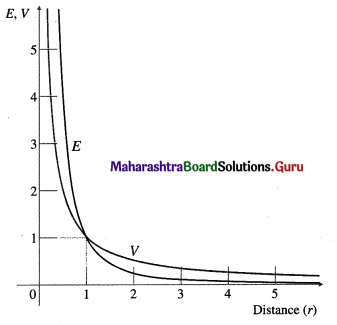Use your brain power (Textbook Page No.194)

Question 1.
Is electrostatic potential necessarily zero at a point where electric field strength is zero? Justify.
Electric potential is a scalar quantity while electric field intensity is a vector quantity. When we add potentials at a point due two or more point charges, the operation is simple scalar addition along with the sign of V, determined by the sign of the q that produces V. At a point, the net field is the vector sum of the fields due to the individual charges. Midway between the two charges of an electric dipole, the potentials due to the two charges are equal in magnitude but opposite in sign, and thus add up to zero. But the electric fields due to the charges are equal in magnitude and direction-towards the negative charge-so that the net field there is not zero. But midway between two like charges of equal magnitudes, the potentials are equal in magnitude and have the same sign, so that the net potential is nonzero. However, the fields due to the two equal like charges are equal in magnitude but opposite in direction, and thus vectorially add up to zero.Do you know (Textbook Page No. 203)

Question 1.
If we apply a large enough electric field, we can ionize the atoms and create a condition for electric charge to flow like a conductor. The fields required for the breakdown of dielectric is called dielectric strength.
In a sufficiently strong electric field, the molecules of a dielectric material become ionized, allowing flow of charge. The insulating properties of the dielectric breaks down, permanently or temporarily, and the phenomenon is called dielectric breakdown. During dielectric breakdown, electrical discharge through the dielectric follows random-path patterns like tree branches, called a Lichtenberg figure. Dielectric strength is the voltage that an insulating material can withstand before breakdown occurs. It usually depends on the thickness of the material. It is expressed in kV/mm. For example, the dielectric strengths of air, polystyrene and mica in kV/mm are 3, 20 and 118. Higher dielectric strength corresponds to better insulation properties.

Remember this (Textbook Page No. 205)

Question 1.
Series combination is used when a high voltage is to be divided on several capacitors. Capacitor with minimum capacitance has the maximum potential difference between the plates.
Series combination of capacitors

1. Equivalent capacitance is less than the smallest capacitance in series. For several capacitors of given capacitances, the equivalent capacitance of their series combination is minimum.
2. All capacitors in the combination have the same charge but their potential differences are in the inverse ratio of their capacitances.
3. Series combination of capacitors is sometimes used when a high voltage, which exceeds the breakdown voltage of a single capacitor, is to be divided on more than one capacitors. Capacitive voltage dividers are only useful in AC circuits, since capacitors do not pass DC signals.

Parallel combination of capacitors

1. For several capacitors of given capacitances, the equivalent capacitance of their parallel combination is maximum.
2. The same voltage is applied to all capacitors in the combination, but the charge stored in the combination is distributed in proportion to their capacitances. The maximum rated voltage of a parallel combination is only as high as the lowest voltage rating of all the capacitors used. That is, if several capacitors rated at 500 V are connected in parallel to a capacitor rated at 100 V, the maximum voltage rating of the capacitor bank is only 100 V.
3. Parallel combination of capacitors is used when a large capacitance is required, i.e., a large charge is to be stored, at a small potential difference.Remember this (Textbook Page No. 207)

Question 1.
(1) If there are n parallel plates then there will be (n-1) capacitors, hence
C = (n – 1) $$\frac{A \varepsilon_{0}}{d}$$
(2) For a spherical capacitor, consisting of two concentric spherical conducting shells with inner and outer radii as a and b respectively, the capacitance C is given by
C = 4πε0$$\left(\frac{a b}{b-a}\right)$$
(3) For a cylindrical capacitor, consisting of two coaxial cylindrical shells with radii of the inner and outer cylinders as a and b, and length l, the capacitance C is given by
C = $$\frac{2 \pi \varepsilon_{0} \ell}{\log _{e} \frac{b}{a}}$$
2. A cylindrical capacitor consists of a solid cylindrical conductor of radius a is surrounded by coaxial cylindrical shell of inner radius b. The length of both cylinders is L, such that L is much larger than b – a, the separation of the cylinders, so that edge effects can be ignored. The capacitance of the capacitor is C = $$\frac{2 \pi \varepsilon_{0} L}{\log _{e}(b / a)}$$.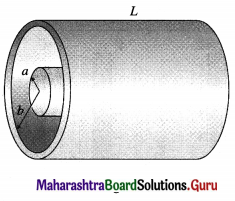3. A spherical capacitor which consists of two concentric spherical shells of radii a and b. The capacitance of the capacitor is C = 4πε0$$\left(\frac{a b}{b-a}\right)$$